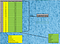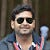# Let’s make weak learner, a strong learner

Let’s start with a small story, which you must have heard in your childhood.

Once upon a time, an old man lived with his three sons in a village. They quarreled all the time.

Months passed by and the old man fell sick. He told his sons to stay united, but they didn’t listen to him. So, he decided to teach them a practical lesson so that they would never forget their differences and stay united.

The old man called his sons. He told them, “I will give you a bundle of sticks. Separate each stick and you will have…

# Precision/Recall Tradeoff

Let’s understand the Precision and Recall before understanding the concept precision/recall tradeoff. To understand the precision and recall, let’s understand the Confusion Matrix first.

# Confusion Matrix

This is the most important performance measure tools for Classification in machine learning. As we know, Classification is one of the supervised tasks in machine learning, where you provide the labeled data to the model or algorithm (Labeled data — Where you have the output or target or class against the features or input, ex shown in below table).Labeled Data

In real time scenario, data will not be like this. We have to collect the data and…

# Correlation:

Correlation explains how one or more variables are related to each other. These variables can be input data features which have been used to forecast our target variable.

Correlation, statistical technique which determines how one variables moves/changes in relation with the other variable. It gives us the idea about the degree of the relationship of the two variables. It’s a bi-variate analysis measure which describes the association between different variables. In most of the business it’s useful to express one subject in terms of its relationship with others.

For example: No of testing vs no of positive cases in Corona.

# Linear Regression in Machine Learning

There are two types of supervised machine learning algorithms or task: Regression and classification.

• Classification — Classification is a process of categorizing a given set of data into classes, it can be performed on both structured or unstructured data. The process starts with predicting the class of given data points. The classes are often referred to as target, label or categories.

Example — Spam, Check defaulter in loan applicant.

• Regression — It predicts the continuous value based on historical data. It Predict the future values on the basis of historical data. …

# Classification topics covered in this story are:

· What is Classification?

· Why do we need classification?

· Classification terminologies

· Types of Classification Algorithms

· Performance measure for classification algorithm

· Algorithm Selection

# 1-> What is Classification?

Two of the most Supervised learning algorithm tasks are Regression (predicting some value) and Classification (Predicting Class). It can be performed on both structure and unstructured data. The process start with predicting the class are often referred to as target, label or category.

In machine learning, classification refers to a predictive modeling problem where a class label is predicted for a given example of input data. Examples of classification problems include, classify if…

# Visualization of Data

Data visualization is the graphical representation of information and data. By using visual elements like charts, graphs, and maps, data visualization tools provide an accessible way to see and understand trends, outliers, and patterns in data.

Python Matplotlib. matplotlib.pyplot is a plotting library used for 2D graphics in python programming language. It can be used in python scripts, shell, web application servers and other graphical user interface toolkits.

When you get huge size of data, it is difficult to see the data or Dataframe using Pandas Library. Hence, we need graph to represent the data, even if you are representing…

# StandardScaler and Normalization with code and graph

Algorithm Selection Process: Below is the algorithm selection process, where we read the data 1st and then we explore the data by various techniques, once data is ready, we divide the data into two parts, Training Data and Testing Data. We train model using training data and we evaluate the model on testing data. At the end we verify the accuracy of each model and best accurate model is used for production

Data PreProcessing: Below are the techniques to preprocess the data prior to feeding the data to model. In Data Transformation, we have Feature scaling. …

## Comprehensions in Python Comprehensions in Python provide us with a short and concise way to construct new sequences (such as lists, set, dictionary etc.) using sequences which have been already defined. Python supports the following 4 types of comprehensions: 1-> List Comprehensions 2-> Dictionary Comprehensions 3-> Set Comprehensions 4-> Generator Comprehensions

1-> List Comprehensions:
output_list = [output_exp for var in input_list if (var satisfies this condition)]

my_list = [1, 2, 3, 4, 4, 5, 6, 7, 7]

evn_numbers = [n for n in my_list if n%2 == 0] # Accept only even number from my_list.
print(evn_numbers)
print([i*2 for i in range(1,10)]) # print twice of the number in range between 1 to 10

2-> Dictionary Comprehensions:
Extending the idea of list comprehensions, we can also create a dictionary using dictionary comprehensions.
The basic structure of a dictionary comprehension looks like below. …